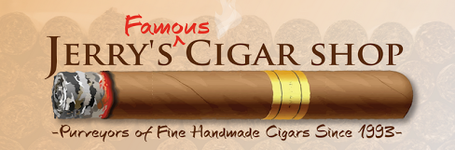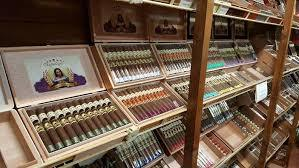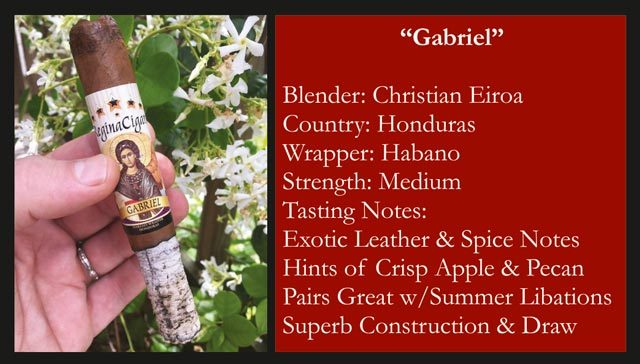/* styles */ We Are Taking Well Studied, Incremental Steps To Try And Return To Some Sense Of Normalcy. This Thoughtful & Methodical Approach Will Make Sure You Are Able To Enjoy Your Cigars With The Highest Level of Confidence In Our Products & Facilities!## BEVERAGE SALES ARE BACK!*

 /* styles */ Beer, Wine & Soda Are Back at the Kerry Forest Shop
 table div table+table+table+table+table+table div table{width:100%;padding:0}table div table+table+table+table+table+table div table img{width:96.23%;padding:0;float:none}table div table+table+table+table+table+table div table td{width:100%;padding:0 1.88% 18px}/* styles */## RESTROOM AVAILABLE*

 /* styles */ Restroom Now Available At the Kerry Forest Pkwy Shop. Bathroom Has Been Fitted for Urinal Service Only.
 table div table+table+table+table+table+table+table+table+table div table{width:100%;padding:0}table div table+table+table+table+table+table+table+table+table div table img{width:96.23%;padding:0;float:none}table div table+table+table+table+table+table+table+table+table div table td{width:100%;padding:0 1.88% 18px}/* styles */## WE'RE ACCEPTING CASH AGAIN*

 /* styles */ Since the Exchange of Cash Is A Major Spreader Of Germs We Can Only Give Change Back in Jerry's Bucks. This Way We Can Ensure That We Are Giving Out Sanitized Change, Something We Can't Control In The Normal Exchange of Cash Bills & Coins. *Example: Customer has a \$38 Cigar Purchase & Tenders \$40 In Cash, Then Is Given \$2 In Jerry's Bucks as Change.
 table div table+table+table+table+table+table+table+table+table+table+table+table div table{width:100%;padding:0}table div table+table+table+table+table+table+table+table+table+table+table+table div table img{width:96.23%;padding:0;float:none}table div table+table+table+table+table+table+table+table+table+table+table+table div table td{width:100%;padding:0 1.88% 18px}/* styles */## OPEN LATER ON WEEKENDS*

 /* styles */ SHARER ROAD STORE: MONDAY - SATURDAY 11am - 6pm * KERRY FOREST PARKWAY STORE: MONDAY - THURSDAY 11am - 6pm FRIDAY & SATURDAY 11am - 9pm* Doors Will Be Locked Promptly at Closing Time.
 table div table+table+table+table+table+table+table+table+table+table+table+table+table+table+table div table{width:100%;padding:0}table div table+table+table+table+table+table+table+table+table+table+table+table+table+table+table div table img{width:96.23%;padding:0;float:none}table div table+table+table+table+table+table+table+table+table+table+table+table+table+table+table div table td{width:100%;padding:0 1.88% 18px}/* styles */## \$5324 ALREADY RAISED FOR LOCAL FOOD BANK RELIEF EFFORTS!HOW YOU CAN HELP...
For every Regina Cigar you buy, we will donate 20% of the sales price to local food banks to assist their outreach to those hurting from this crisis.

 table div table+table+table+table+table+table+table+table+table+table+table+table+table+table+table+table+table+table div table{width:100%;padding:0}table div table+table+table+table+table+table+table+table+table+table+table+table+table+table+table+table+table+table div table img{width:96.23%;padding:0;float:none}table div table+table+table+table+table+table+table+table+table+table+table+table+table+table+table+table+table+table div table td{width:100%;padding:0 1.88% 18px}/* styles */## REGINA OF THE MONTH/* styles */ This month we are featuring the Amazing Regina "Gabriel" Get a \$10 Jerry's Gift Card for every 4 Pack you buy! That works out to almost a 30% Discount! And 20% of your purchase goes to Local Food Banks! THIS 4 PACK SELLS FOR \$35.80 + tax ...and you get \$10 back in Jerry's Bucks ~SECURE ONLINE CHECKOUT~ Available for In Store Pickup or Free Shipping!
 table div table+table+table+table+table+table+table+table+table+table+table+table+table+table+table+table+table+table+table+table+table+table+table div table{width:100%;padding:0}table div table+table+table+table+table+table+table+table+table+table+table+table+table+table+table+table+table+table+table+table+table+table+table div table img{width:96.23%;padding:0;float:none}table div table+table+table+table+table+table+table+table+table+table+table+table+table+table+table+table+table+table+table+table+table+table+table div table td{width:100%;padding:0 1.88% 18px}/* styles */# WHAT STAYS THE SAME AT THE SHOPS:

▪ Front Door & Curbside Service
▪ Locker Access, One Patron at a Time
▪ Humidors Remain Safely Sealed From Contamination
▪ Outdoor Smoking at Kerry Forest Shop
▪ Bring Your Own Folding Chair
▪ Pre-Marked 6 Foot Spacing Outside
▪ Free UPS Shipping On Orders >\$29
 ▪ Front Door & Curbside Service
 ▪ Locker Access, One Patron at a Time
 ▪ Humidors Remain Safely Sealed From Contamination
 ▪ Outdoor Smoking at Kerry Forest Shop
 table div table+table+table+table+table+table+table+table+table+table+table+table+table+table+table+table+table+table+table+table+table+table+table+table+table div table{width:100%;padding:0}table div table+table+table+table+table+table+table+table+table+table+table+table+table+table+table+table+table+table+table+table+table+table+table+table+table div table img{width:96.23%;padding:0;float:none}table div table+table+table+table+table+table+table+table+table+table+table+table+table+table+table+table+table+table+table+table+table+table+table+table+table div table td{width:100%;padding:0 1.88% 18px}/* styles */table div table+table+table+table+table+table+table+table+table+table+table+table+table+table+table+table+table+table+table+table+table+table+table+table+table+table+table div table{width:100%;padding:0}table div table+table+table+table+table+table+table+table+table+table+table+table+table+table+table+table+table+table+table+table+table+table+table+table+table+table+table div table img{width:96.23%;padding:0;float:none}table div table+table+table+table+table+table+table+table+table+table+table+table+table+table+table+table+table+table+table+table+table+table+table+table+table+table+table div table td{width:100%;padding:0 1.88% 18px}/* styles */